# JP4102187B2 - How to align a grid of points according to the features of a digital image - Google Patents

How to align a grid of points according to the features of a digital image Download PDF

## Info

Publication number
JP4102187B2
JP4102187B2 JP2002548679A JP2002548679A JP4102187B2 JP 4102187 B2 JP4102187 B2 JP 4102187B2 JP 2002548679 A JP2002548679 A JP 2002548679A JP 2002548679 A JP2002548679 A JP 2002548679A JP 4102187 B2 JP4102187 B2 JP 4102187B2
Authority
JP
Japan
Prior art keywords
image
grid
points
function
method
Prior art date
Legal status (The legal status is an assumption and is not a legal conclusion. Google has not performed a legal analysis and makes no representation as to the accuracy of the status listed.)
Expired - Fee Related
Application number
JP2002548679A
Other languages
Japanese (ja)
Other versions
JP2004533026A (en
Inventor
ヘール，アイラ・デイビッド
Original Assignee
ランドマーク・グラフィックス・コーポレーション
Priority date (The priority date is an assumption and is not a legal conclusion. Google has not performed a legal analysis and makes no representation as to the accuracy of the date listed.)
Filing date
Publication date
Priority to US25434700P priority Critical
Priority to US09/818,220 priority patent/US6631202B2/en
Application filed by ランドマーク・グラフィックス・コーポレーション filed Critical ランドマーク・グラフィックス・コーポレーション
Priority to PCT/US2001/048239 priority patent/WO2002047030A2/en
Publication of JP2004533026A publication Critical patent/JP2004533026A/en
Application granted granted Critical
Publication of JP4102187B2 publication Critical patent/JP4102187B2/en
Application status is Expired - Fee Related legal-status Critical
Anticipated expiration legal-status Critical

## Images

•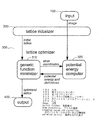•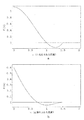•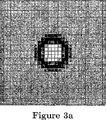•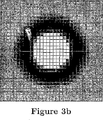•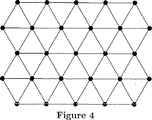•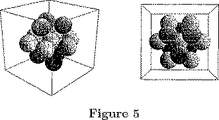•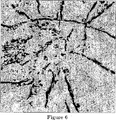•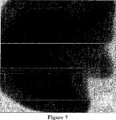•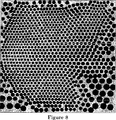•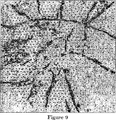•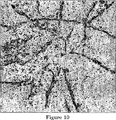•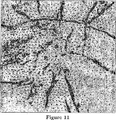•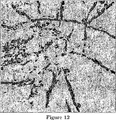•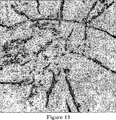•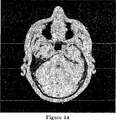•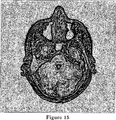•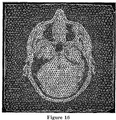•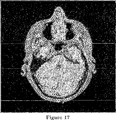•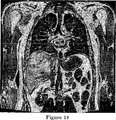•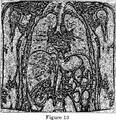•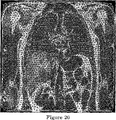••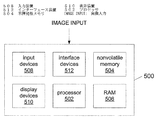•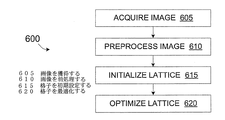## Classifications

• GPHYSICS
• G06COMPUTING; CALCULATING; COUNTING
• G06TIMAGE DATA PROCESSING OR GENERATION, IN GENERAL
• G06T17/00Three dimensional [3D] modelling, e.g. data description of 3D objects
• G06T17/20Finite element generation, e.g. wire-frame surface description, tesselation

## Description


Cross-reference of related applications
Not applicable.

background
Field of Invention
The present invention relates to the analysis of digital images, and more particularly to building a computational mesh from such images.

Description of prior art
Digital images are often analyzed to obtain a mesh for further computation. For example, seismic images are analyzed to obtain a geological mesh that is used to simulate the oil flow in an underground reservoir. Similarly, medical images of the human brain are analyzed to obtain a mesh that is used to simulate intra-arterial blood flow. A slightly different example is image morphing that can use a mesh derived from one image to efficiently encode the differences in subsequent images. In all these applications, the image is analyzed to build a mesh.

Today's images and meshing
Specifically, such applications often consist of the following sequence of steps:
(1) Process the image to enhance specific features.
(2) Find curves or surfaces in the image that join specific areas.
(3) The space determined by these areas is filled with a calculation mesh.
(4) Simulate several processes on the space-filling mesh.

For applications of medical image processing, see Cebral, J. et al. R. And Loehner R.M. Written by From Medical Images to CFD Meshes, Proceedings of the 8 th International Meshing Roundtable, p. 321-331, Sandia, 1999 ("From Medical Images to CFD Meshes" Proceedings of the 8th International Meshing Roundtable, pages 321-331, Sandia, 1999). For applications of seismic image processing, see Garrett, S .; , Griesbach, S .; Johnson, D .; Jones, D .; Lo, M .; , Orr, W .; , And Word, C.I. Author, Earth Model Synthesis, First Break, v. 15, no. 1, p. 13-20, 1997 ("Ground Model Synthesis, Initial Movement" Vol. 15, No. 1, pp. 13-20, 1997).

For various reasons, each step of this sequence is often performed independently today with little need for subsequent steps. For example, in step (2) it is common to generate a coupling curve or surface with more details than can be represented by the space-filling mesh used in step (4). Seismic reflections representing geological boundaries are usually mapped at a higher resolution than can actually be used with meshes for oil layer simulation. This leads to the “scale-up” or “upscaling” problem (1997) cited by Garett et al.

This discrepancy in resolution is often accompanied by a discrepancy in data structure. For example, a two-dimensional (2D) curve represented by a simple linked list of line segments in step (2) may be a relatively complex mesh of triangles in step (3). Such discrepancies can disrupt analysis sequences today and can cause discrepancies between the image processed in step (1) and the mesh used in step (4), both of which are difficult to quantify. There is sex.

Interruption is costly. In the example of seismic image analysis, it may take one month today to repeat this sequence once. Because of this great sacrifice, it is difficult to perform multiple iterations to evaluate the uncertainty of the analysis results.

One reason for inconsistencies in resolution and data structure is in the execution of analysis sequences. Historically, the four steps listed above have been performed by separate computer programs, developed independently, and used by experts in different fields. Today, one person or one small group may perform all four steps of this sequence, so there is a need to better integrate these steps.

Another reason for the contradiction is lack of methodology. The simulation step (4) often requires a numerical solution of the partial differential equation (PDE). In the past, these solutions could only be performed on structured meshes that could index mesh elements as simple arrays. The conversion from the unstructured mesh generated in step (3) to the structured mesh creates further inconsistencies between the processed image and the mesh used in the simulation. However, recent advances in numerical methods for solving PDEs on unstructured meshes suggest that this transformation and the resulting inconsistencies can be avoided.

Mesh by lattice
The accuracy of most calculations performed on meshes depends on the regularity (sometimes regular) nature of the mesh elements. Simulations performed on very regular triangular meshes with approximately equilateral triangles are more accurate than simulations performed on irregular meshes with elongated triangles.

This is one reason why Delaunay triangulation is popular. Assuming a set of points represent 2D mesh node positions, the Delaunay triangulation of those points yields the triangle that is closest to the equilateral edge compared to all other possible triangulations. However, the Delaunay triangulation alone does not guarantee a regular mesh. Therefore, it is necessary to carefully select the position of the node of the mesh.

(An overview of 2-D Delaunay triangulation and 3-D Delaunay triangulation applied to the meshing problem is given by Bern, M., and Epstein, D., Mesh Generation and Optimal Triangulation, in Computational in Eucommendation Geometry. , D.-Z., and Hwang., F. K., World Scientific, 1995 ("Mesh generation and optimal triangulation in Euclidean geometry calculations").

In fields other than image analysis, the problem of selecting the optimal mesh node position is well studied. One solution to this problem is based on the observation that the triangulation of a set of points defined by a simple crystal lattice results in a very regular mesh. (Mello, U. T., and Cavaranti, P. R., A Point Creation Strategies for Mesh Generation Using Crystal Lattice as Templates, Proceedings of the 9 th International Meshing Roundtable, p. 253-261, Sandia, 2000 ("Mesh generation point generation strategy using crystal lattice as template", Proceedings of the 9th International Meshing Roundtable, pp. 253-261, Sandia, 2000). However, a uniform grid of points can rarely align exactly with the boundaries of the object being meshed. Thus, some solutions start with a nearly uniform grid and then the physical force between atoms or bubbles to automatically move the mesh nodes (atoms or bubbles) to a more optimal position. Use a numerical model. (Shimada, K., Physically-Based Mesh Generation: Automated Triangulation of Surfaces and Volumes via Bubble Packing, Ph, D. thesis, Massachettes. Surface and volume triangulation by bubble packing "), Shimada, K. and Gossard, DC, Bubble Mesh: Automated Triangular Meshing of Non-Manifold Geometry by Sphere Packing. roses of the Third Symposium on Solid Modeling and Applications, p. 409-419, 1995 ("Bubble mesh: automatic triangulation of non-manifold shapes by sphere packing" Proceedings of the 3rd Symposium on Solid Modeling and Equipment, 409-419 (1995)) 2000 Itoh, T .; Inoue, K .; Yamada, A .; , And Shimada, K .; Et al., US Pat. No. 6,124,857, named Meshing Methods and Apparatus, extends this work to quadrilateral meshing. Bossen, F.M. J. et al. , And Heckbert, P .; S. Written by A Pliant Method for Anisotropic Mesh Generation, Proceedings of the 5 th International Meshing Roundtable, p. 63-74, Sandia, 1996 (see also “Adaptive Method for Anisotropic Mesh Generation” Proceedings of the 5th International Meshing Roundtable, pages 63-74, Sandia, 1996).

In all of these examples, grid-based meshing begins with a geometric model that precisely defines the inner and outer boundaries of the object to be meshed. Accordingly, there is a need for a meshing system and method that can address the problem when such boundaries cannot be known in advance.

[Patent Document 1]
U.S. Pat. No. 4,908,781
1990, Levinthal, C .; , And Fine, R .; Computing Device for Calculating Energy and Pairwise Central Forces of Particle Interactions.
[Patent Document 2]
US Pat. No. 5,596,511
In 1997, Toyoda, S .; Ikeda, H, Hshimoto, E .; , And Miyakawa, N .; Computing Method and Apparatus for a Many-Body Problem.
[Patent Document 3]
US Pat. No. 6,124,857
2000 Itoh, T .; Inoue, K .; Yamada, A .; And Shimada, K .; Named Meshing Method and Apparatus
[Non-Patent Document 1]
Bentley, J .; L. And Friedman, J .; H. , Data Structures for Range Searching, ("Data Structure for Range Search") Computing Surveys, vol. 11, no. 4,1979
[Non-Patent Document 2]
Bern, M.M. And Epstein, D.M. Author, Mesh Generation and Optimal Triangulation, in Computing in Euclidian Geometry, Du, D .; -Z. And Hwang. , F.A. K. Edit. , World Scientific, 1995 ("Mesh generation and optimal triangulation in Euclidean geometry calculation")
[Non-Patent Document 3]
Bossen, F.M. J. et al. , And Hechbert, P .; S. Written by A Pliant Method for Anisotropic Mesh Generation, Proceedings of the 5 th International Meshing Roundtable, p. 63-74, Sandia, 1996 ("Adaptive Method for Anisotropic Mesh Generation" Proceedings of the 5th International Meshing Roundtable, 63-74, Sandia, 1996)
[Non-Patent Document 4]
Byrd, R.A. H. Nocedal, J .; , And Schnabel, R .; B. Author, Representations of Quasi-Newton Matrices and Their Use in Limited Memory Methods, Technical Report NAM-03, Northwestern University, Department of Electrical Engineering and Computer Science, 1996 (Northwestern University, Electrical Engineering and Computer Science Department, Technical Report NAM-03, 1996 "Representations of quasi-Newton matrices and their use in limited memory methods")
[Non-Patent Document 5]
Cebral, J. et al. R. And Lohner, R .; Written by From Medical Images to CFD Meshes, Proceedings of the 8 th International Meshing Roundtable, p. 321-331, Sandia, 1999 ("From Medical Images to CFD Meshes" Proceedings of the 8th International Meshing Roundtable, pp. 321-331, Sandia, 1999)
[Non-Patent Document 6]
Garrett, S.M. , Griesbach, S .; Johnson, D .; Jones, D .; Lo, M .; , Orr, W .; , And Word, C.I. Author, Earth Model Synthesis, First Break, v. 15, no. 1, p. 13-20, 1997 ("Surface Model Synthesis, First Movement" Vol. 15, No. 1, pp. 13-20, 1997)
[Non-Patent Document 7]
Mello, U .; T.A. , And Cavalcanti, P.M. R. Written by A Point Creation Strategies for Mesh Generation Using Crystal Lattice as Templates, Proceedings of the 9 th International Meshing Roundtable, p. 253-261, Sandia, 2000 (“Mesh generation point generation strategy using crystal lattice as template”, Proceedings of the 9th International Meshing Roundtable, pp. 253-261, Sandia, 2000)
[Non-Patent Document 8]
Shimada, K .; Physically-Based Mesh Generation: Automated Triangulation of Surfaces and Volumes Via Bubble Packing, Ph.D. thesis, Massachusetts Institute of Technology, 1993 (Doctoral Dissertation, Massachusetts Institute of Technology 1993 "Physics-Based Mesh Generation: Automatic Triangulation of Surfaces and Volumes with Bubble Packing")
[Non-patent document 9]
Shimada, K .; And Gossard, D .; C. Written by Bubble Mesh: Automated Triangular Meshing of Non-Manifold Geometry by Sphere Packing, Proceedings of the Third Symptom on Solid Amploid Solid Aid. 409-419, 1995 ("Bubble mesh: automatic triangulation meshing of non-manifold shapes by sphere packing" Proceedings of the 3rd Symposium on Solid Modeling and Equipment, ACM Publishing, 409-419, 1995)

Summary of the Invention
A method for generating a grid of points for features in a digital image, according to various embodiments of the present invention, includes a process for initializing the grid and a process for optimizing the alignment of the grid with respect to image features. The optimization process operates by adjusting the points of the grid in order to extremize the synthesis function of the spatial coordinates of the grid points. The composite function is weighted with a first function of the paired distance between points and a second function derived from the image (eg, calculated from sampled values of the image near the grid points). Combination.

The mesh can be generated from an optimized grid. The mesh can be used in any of a variety of applications. For example, the mesh can be used to simulate the process associated with the image. As another example, a mesh can be used to compress an image of a sequence of images (eg, a video stream) or subsequent images.

In some implementations, the optimized grid can be used to encode information associated with the image with or without the generation of an intermediate mesh. Any of various types of related information is contemplated.

Purpose and benefits
One object of at least some embodiments of the present invention is a method that allows the above image analysis sequence (prior art description) to be replaced with the following more efficient sequence.
(1) Process the image to enhance specific features.
(2) Fill the space with computational mesh aligned to the image features.
(3) Simulate several processes on the space-filling mesh.
Rather than finding the boundaries of regions in the image and meshing those regions, you just build a mesh aligned with the boundaries.

A further object of some embodiments of the invention is to use this method to construct:
A very regular mesh suitable for further calculations such as solving partial differential equations.
Graded meshes where the density of the nodes in the mesh depends on the complexity of the image or a user-specified function.
3D mesh that can use 3D images.

These and other objects and advantages of various embodiments of the present invention will become more apparent upon review of the following description and drawings.

While the invention is susceptible to various modifications and alternative forms, specific embodiments thereof are shown by way of example in the drawings and are described in detail below. However, the drawings and detailed description thereof are not intended to limit the invention to the particular forms disclosed, but are intended to embrace all variations that fall within the spirit and scope of the invention as defined by the appended claims. It is to be understood that this is intended to cover equivalent forms and alternative forms.

Detailed Description of the Preferred Embodiment
This document describes a method for generating a grid of points for features in a digital image. The method includes initializing and optimizing a grid of points. In one set of embodiments, the points of the optimized grid tend to align (match) with the features of the image. In a second set of embodiments, the optimized grid points tend to align along the features rather than on the features of the image. In other embodiments, the optimized grid points may tend to align with certain features in one image but not with other features.

Below, the points of the grid are called atoms. The process for initial setting of the lattice will be described with reference to the atom structure of the crystal. Similarly, lattice optimization is described with respect to minimizing the potential energy of the atom lattice. Although these physical models make it easier to understand our explanation, the atoms in the present invention are just one point.

FIG. 1 shows in more detail the data flow between the main components of the method. Data input component 100 generates an image that has already been processed elsewhere to highlight specific features. Here, the image is obtained directly as a result of such processing or loaded from the memory of the computer. The grid initializer 200 creates an initial grid of atoms that spans the entire image. The grid optimizer 300 moves the atom so that the grid aligns with the features of the image. The data output component 400 passes the optimized atom grid to another process or stores it in the memory of the computer to consume the optimized atom grid.

The lattice optimizer 300 includes a generic function minimizer 310 and a potential energy computer 320. The generic minimizer 310 iteratively searches for a minimal function of many variables. During the search, the minimizer needs to iteratively compute the function and its partial derivative for each variable. Here, the variable is a spatial coordinate of the atom of the lattice. Given an image with atom coordinates, the potential energy computer 320 calculates the potential energy of the lattice and its partial derivative with respect to the coordinates of each atom.

Calculation of potential energy
An atom in a two-dimensional (2-D) space has an x coordinate and ay coordinate, and an atom in a three-dimensional (3-D) space has an x coordinate, ay coordinate, and a z coordinate. The vector x indicates the x space coordinate and y space coordinate (or x, y, z) of a point in 2-D (or 3-D) space. Position x i And x j Given two atoms of | x i -X j | Indicates the distance between them. In the preferred embodiment, the vector norm | · | used to calculate this inter-atom distance is the Euclidean norm. However, any of a variety of other norms can be used instead.

Paired potential functions
In terms of computational efficiency, we have determined by a pair of force functions so that the total force exerted on one atom by its surrounding atoms is simply the sum of the forces exerted by each of the surrounding atoms. Model the interaction between atoms. Although simplified in this way, there are many suitable options for the paired force function.

In order not to have a plurality of atoms at the same or substantially the same coordinates, when the atoms are too close to each other, the force between the atoms may be a repulsive force (positive). Similarly, in order not to generate a large space without atoms, when the atoms are too far apart, the force between the atoms may be attractive (negative). To facilitate numerical calculations, forces can be bounded. To prevent the atoms in the grid from exerting a force on all other atoms, the force may be zero beyond the cut-off distance. Furthermore, the force function may be continuous depending on the distance between the atoms. The force function proposed by Shimada (1993) has these properties. Thus, in one set of embodiments, we use the Shimada force function as described below. However, any of a variety of force functions consistent with their nature can be used.

Let d be the nominal distance between the two atoms, which is the distance at which the force transitions from repulsive force to attractive force between the two atoms. In this case, x i And x j The force f between two atoms arranged at can be obtained by a cubic polynomial.
[Expression 1]
Where u is
[Expression 2]
Is the normalized distance between two atoms defined by. The coefficients of this polynomial function ensure that the force is bounded and continuous, the force is zero for u = 1 and u ≧ 3/2, positive for 0 ≦ u <1, and 1 < It is guaranteed that u <3/2 is negative. FIG. 2a shows this force function.

In general, forces related to atoms are vectors. Here, the direction of this vector is the sign of f (u) and the position x of two atoms. i And x j It shows with.

It is more convenient to work with scalar potential than to work with multiple components of vector force. Thus, according to well-known conventions, we define this force as the negative of the slope of the scalar potential.
[Equation 3]
The integration constant 153/256 is selected so that φ (u) is continuous at u = 3/2. FIG. 2b shows this potential function. As expected, the potential function φ (u) has a minimum value at the normalized distance u = 1. There, the force function f (u) is zero.

Potential energy and potential field
Given the normalized distance u potential function φ (u), we define the atomic potential energy as the sum of the paired potentials shown below.
[Expression 4]
Where x 1 , X 2 ,. . . , X n Is the coordinates of n atoms in the lattice, and d (x) is the nominal inter-atom distance function at position x. The nominal distance function d (x) need not be a constant, but in order to ensure a smooth gradient grid we need that function to be smooth. Specifically, we have | x i -X j D (x for | / d i ) ≒ d (x j ) Is smaller than the cut-off distance 3/2 of the potential function φ (u). < Requires <1. In this case, the factor 1/2 is 2 degrees φ [| x in the definition of the total potential energy A i -X j | / D (x j )] ≒ φ [| x j -X i | / D (x i )] Is compensated for.

The inventors also define the atomic potential energy A by an atomic potential field equation.
[Equation 5]
Therefore,
[Formula 6]
It is. That is, atomic potential energy is defined as half the sum of the values obtained by evaluating the atomic potential field in the coordinates of the atom.

Similarly, the inventors have calculated the image potential energy.
[Expression 7]
It is defined as Here, b (x) is an image potential field based on the input image.

In many situations, the image potential field is simply an image (or a smoothed version of the image) and is typically represented by a number of 2-D (or 3-D) arrays stored in computer memory. The Here, in order to emphasize the similarity between the atomic potential field and the image potential field (and for later use), we use the term “potential field”.

In one set of embodiments, the image potential field takes a minimum value (eg, b (x) ≈−1) within a particular feature and a maximum value (eg, b (x) ≈0) in a non-target region. It is assumed that the image is processed so that In this case, minimizing the image potential energy B is equivalent to moving the atom to the minimum corresponding to a particular feature of the image.

In a second set of embodiments, the image potential field takes a maximum value (eg, b (x) ≈1) within a particular feature and a minimum value (eg, b (x) ≈0 in non-target areas. ) Is assumed to have been processed. In this case, minimizing the image potential energy B is equivalent to moving the atom away from the maximum corresponding to a particular feature of the image.

In a third set of embodiments, the image potential field has a maximum value (eg, b (x) ≈1) along the first subset of image features, along the second subset of image features. Minimum values (eg, b (x) ≈−1) and intermediate values (eg, b (x) ≈0) away from the image features.

More generally, we move the atom to minimize the weighted sum of atomic potential energy and image potential energy as shown below.
P = P (x 1 , X 2 ,. . . , X n ) ≡ (1-β) A + βB (4)
We call this total potential energy. The magnification β determines the relative contribution of A and B to the total potential energy P. If β = 0, the atom tilts into a perfectly regular grid that is not aligned with the image. When β = 1, the atom reacts only to the image sample value. That is, the atom moves to the minimum value of the image only based on the attractive force and / or moves from the maximum value of the image only based on the repulsive force. Thus, atoms tend to gather at the minimum value in the image and to retreat from the maximum value, resulting in a very irregular lattice. In a typical case, we choose β≈1 / 2 to obtain a nearly regular grid and a grid that takes into account image features.

The total potential energy represented by the potential fields a (x) and b (x) is
[Equation 8]
It is. Total potential field
p (x) ≡ (1-β) α (x) + βb (x) (5)
The total potential energy is
[Equation 9]
It is.

Similar to the nominal distance function d (x), the magnification β in the equations (5) and (6) may be a function that smoothly changes the position x. (For d (x), smoothness means that the derivative of β (x) is negligible.) This generalization allows lattice regularity and response to image features (eg, to image features). Balance of repulsive force or repulsive force from image features) can be spatially varied. The response of the grid to image features may be more important in some parts of the image than in other parts. In the present specification, for the sake of simplification, the inventors indicate a constant magnification by β.

The total potential energy P is the coordinate x of an atom with many local minima. 1 , X 2 ,. . . , X n Is a non-quadratic function of (Note that, for example, swapping the coordinates of any two atoms does not change P.) Therefore, any search for a minimum value, typically a value close to the coordinates of the initial grid, is not possible. Need to iterate. An efficient iterative search requires the partial derivative of P to be iteratively calculated with respect to the coordinates of the atom. For example, assume that P has changed with respect to the x coordinate of the i th atom.
[Expression 10]
Partial differential of atomic potential energy ∂A / ∂x i The term φ [| x i -X j | / D (x j )] ≒ φ [| x j -X i | / D (x i We recall that the)] appears twice in the double sum of equation (1). Therefore,
[Expression 11]
It is. Similar results can be easily obtained for partial differentiation with respect to the y coordinate of each atom (and z coordinate in 3-D).

Calculation
Calculation of the total potential energy P requires calculation of its components A and B. To calculate the image potential energy B according to equation (3), the atom position x = x i For each image potential field b (x) needs to be calculated. Images are usually sampled uniformly. b (x i The simplest and most efficient approximation to i Is the value of the image potential field b (x) in the image sample closest to. Although more precise approximation (interpolation) is possible, we have used this simple and fast nearest-neighbor interpolation in all of the embodiments presented herein. Equation (3) indicates that the cost (computation amount) of calculating B is O (n). Here, n is the number of atoms.

Conversely, the double sum in equation (1) is the most obvious way to calculate A is O (n 2 ). In an actual application example, O (n for calculating A 2 N is large enough so that the cost is much larger than the O (n) cost to get B. In order to reduce the cost of calculating A, we have the potential function φ (u) of zero for a normalized distance u greater than the cutoff distance 3/2. Design can be utilized. Position x = x i Only the atom closest to the atom located at is the atomic potential field a (x i ).

This observation is x = x i The distance of each atom arranged at 3 / 2d (x i ) Leads to the problem of determining which nearby atoms are in. The solution to this problem is non-trivial. This is because atoms move iteratively during grid optimization.

For example, if you create a list of neighboring atoms, one for each atom, you will need to update these lists each time the atom moves (or at least check if those lists need updating) Need to check for). For a grid with nearly constant density, the cost of constructing and updating such a list using a simple data structure is O (mn). Here, m is the average number of neighboring atoms within the cutoff distance. In the case of a variable density grid, a more complicated data structure is required, and the cost is O (mn · logn). (See, for example, Bentley, JL and Friedman, JH, Data Structures for Range Searching, ("Data Structures for Range Search") Computing Surveys, vol. 11, no. 4, 1979. Also, U.S. Pat. No. 4,908y, 1990, 1997, Levinthal, C., and Fine, R., et al. Computation by S., Ikeda, H, Hshimoto, E., and Miyakawa, N. et al. (See also No. 5,596,511 entitled ing Method and Apparatus for a Many-Body Problem.)

Our equation for the atomic potential energy A with respect to the atomic potential field a (x) shows a simpler solution. We interpret equation (2) as a method for calculating a sampled atomic potential field a (x) as image potential field b (x). Specifically, the inventors express a (x) with a 2-D array or a 3-D array having the same dimensions as the array used to represent the image b (x). First, we initialize a (x) to zero for all sampled x. Then position x = x j For each atom placed in, we have sampled potential function φ [| x−x j | / D (x j )]. This accumulation is at position x j Radius 3 / 2d (x j ) Spatially limited to samples within a circle (or sphere). Here, the contribution of the sampled potential function is not zero.

Figures 3a and 3b show two such potential functions for nominal distances d = 4 and d = 8, respectively. Halftone levels between black and white correspond to sampled function values between -0.05 and 0.05, respectively. The atomic potential field a (x) is an accumulation of many such functions. For computational efficiency, these sampled potential functions are pre-calculated and listed for different nominal distances d. Then, given d (x) for an arbitrary position x, the appropriate value of the potential function can be efficiently determined by a table search (or table search and interpolation).

Preferred embodiment
Our analysis has suggested two very different algorithms for calculating the total potential energy and its partial derivative. The preferred embodiment of the present invention uses equations (2), (5) to calculate the total potential field p (x) and then uses equation (6) to calculate the total potential energy. Equation (10) is used to calculate the partial derivative. The following pseudo code listing illustrates this algorithm in detail.
Algorithm 1: P, ∂P / ∂x i , ∂P / ∂y i And ∂P / ∂z i Calculate
101: Initialize the total potential field p (x) = βb (x)
102: All atom positions x j = X 1 , X 2 ,. . . , X n Regarding {
103: | xx j | <3 / 2d (x j ) For all x like {
104: p (x) = p (x) + (1-β) φ [| x−x j | / D (x j )]
105:}
106:}
107: Initializing the total potential energy P = 0
108: All atom positions x j = X 1 , X 2 ,. . . , X n Regarding {
109: P = P + 1/2 [p (x i ) + Βb (x i )]
110: ∂P / ∂x i = 1/2 [p (x i +1, y i , Z i ) -P (x i -1, y i , Z i )]
111: ∂P / ∂y i = 1/2 [p (x i , Y i +1, z i ) -P (x i , Y i -1, z i )]
112: ∂P / ∂z i = 1/2 [p (x i , Y i , Z i +1) -p (x i , Y i , Z i -1)]
113:}

Rows 101 to 106 calculate the total potential field p (x) sampled as the image potential field b (x). Given this field, rows 107-113 show the total potential energy P and its partial derivative ∂P / ∂x. i , ∂P / ∂y i , ∂P / ∂z i Calculate In line 109, the atom position x i The total potential field and the image potential field at can be approximated by selecting the corresponding field value at the nearest image sample location, as described above, or by any desired interpolation scheme. The partial derivative of rows 110-112 is calculated from the total potential field using a simple centered-finite-difference approximation, but an alternative (eg higher) number for the derivative Approximate values can be used instead. For clarity, this list is a 3-D coordinate space. For 2-D space, z coordinate and partial differential ∂P / ∂z i Is simply ignored.

Assuming that the atom position matches the nominal distance function d (x), the calculation cost of Algorithm 1 is O (N). Here, N is the number of samples in the image. We sample a total potential field such as an image, and a potential function whose atoms are spatially limited to those samples of the total potential field closest to that atom Recall that it gives (similar to FIG. 3). Thus, the cost of accumulating contributions from all atoms is proportional to the number of samples N in that field.

The cost of this algorithm corresponds to the cost of conventional image processing and requires a data structure that is not as complex as a simple array that represents an image. Further, this cost is the same for the non-constant nominal distance function d (x) as for the constant nominal distance d.

Alternative form
An alternative form of the invention does not calculate the total potential field. Instead, this alternative uses equations (1), (3), (4) to calculate the total potential energy and equations (7), (8) to calculate its partial derivatives. , (9) is used. The following pseudo code listing illustrates this algorithm in detail.
Algorithm 2: P, ∂P / ∂x i , ∂P / ∂y i , ∂P / ∂z i Calculate
201: Initialize total potential energy P = 0
202: All atom positions x j = X 1 , X 2 ,. . . , X n Regarding {
203: P = P + βb (x j )
204: ∂P / ∂x i = 1 / 2β [b (x i +1, y i , Z i ) -B (x i -1, y i , Z i )]
205: ∂P / ∂y i = 1 / 2β [b (x i , Y i +1, z i ) -B (x i , Y i -1, z i )]
206: ∂P / ∂z i = 1 / 2β [b (x i , Y i , Z i +1) -b (x i , Y i , Z i -1)]
207: | x i -X j | <3 / 2d (x i ) All atom positions x j Regarding {
208: P = P + 1/2 (1-β) φ [| x i -X j | / D (x i )]
209: Δ = (1−β) φ ′ [| x i -X j | / D (x i )] / [D (x i ) | X i -X j ｜] is calculated
210: ∂P / ∂x i = ∂P / ∂x i + Δ (x i -X j )
211: ∂P / ∂y i = ∂P / ∂y i + Δ (y i -Y j )
212: ∂P / ∂z i = ∂P / ∂z i + Δ (z i -Z j )
213:
214:}

For each atom, the total potential energy P, for each neighboring atom, row 203 accumulates image potential energy B, and row 208 accumulates atomic potential energy A. Similarly, rows 204-206 and rows 210-212 accumulate the contribution of the partial derivative of the image and atomic potential energy to the partial derivative of the total potential energy.

Line 207 is x = x i Is meant to use an auxiliary data structure that allows for the quick determination of the nearest atom that is closest to the atom located at. This data structure needs to be rebuilt or updated in some way each time the atom moves. The atom moves iteratively in any iterative search that calculates atom coordinates that minimize the total potential energy. Therefore, the cost of maintaining this data structure can be enormous. For the most efficient data structure, this cost is higher for the non-constant nominal distance function d (x) than for the constant nominal distance d.

Initial grid settings
As described above, the total potential energy P is a non-quadratic function of the coordinates of the atom with many local minimum values. During grid optimization, we search for the minimum and move the atom iteratively. In practice, we do not seek or find a global minimum. Instead, we find an optimized lattice of atoms that is close to the initial lattice. Therefore, the initial lattice is preferably as follows.
Minimizing atomic potential energy (locally)
Very regular,
It coincides with the nominal distance function d (x).

Constant nominal distance
In the case of a constant nominal distance d, we can easily construct the initial lattice by its nature. FIG. 4 shows such a grid for 2-D space. With this ideal lattice, atoms can be combined to form equilateral triangles. The distance between any atom and its six nearest neighbors is simply a constant nominal distance d. At this distance, the force exerted by any one atom on another atom is exactly zero, and the atomic potential energy is locally minimized.

FIG. 5 shows a regular lattice in the case of 3-D space. This is a face-centered cubic (FCC) lattice, in which the atom is composed of horizontal layers such as the 2-D lattice in FIG. 4, but each layer is slightly filled to fill the holes in the upper and lower layers. Shifted. To make FIG. 5 easier to understand, atoms in different layers are rendered with spheres of different tones. The distance between any atom and its 12 nearest neighbors is equal to the constant nominal distance d.

Contrary to an equilateral triangle (as in FIG. 4) that can completely fill the 2-D space, an equilateral tetrahedron cannot fill the 3-D space. However, in order to obtain a very regular tetrahedron, the atoms of the FCC lattice can be triangular.

Variable nominal distance
The initial construction of an atom is even more difficult in the case of a nominal distance function d (x) rather than a constant.

The first complication is that a function d (x) that would otherwise be specified must be calculated. As an example of a method for calculating this function, an image shown in FIG. 6 (that is, an image potential field b (x)) is assumed. This image is a horizontal slice of a 3D seismic image (not shown) that has been processed to highlight faults or surface geological discontinuities. The feature extending in the black line shape of this image represents the trace of the fault crossing this horizontal slice. The fault is almost vertical and almost perpendicular to this horizontal slice.

FIG. 7 shows the result of smoothing the seismic image to obtain an estimate of the nominal distance function d (x). The darkest area of the drawing corresponds to the minimum distance of d = 6 samples and the brightest area corresponds to the maximum distance of d = 18 samples. These minimum and maximum distances were explicitly specified based on the level of detail found in the seismic image. The distance becomes shorter in the left part from the center of the image and becomes longer in the lower right part. The average distance is about 9.7 samples.

In some applications, the nominal distance function d (x) may be specified explicitly or iteratively constructed using a computer program for image coloring. For the present invention, the actual method of calculating the function d (x) is not important. As noted above, we have found that this function is smooth, i.e., | ∇d | < It only needs to be <1.

The second complexity is to construct a lattice atom that matches the nominal distance function d (x). Here we describe two algorithms for this configuration.

Preferred embodiment
The preferred embodiment of the present invention uses an algorithm for generating a pseudo-regular lattice. The following pseudo code listing illustrates this algorithm in detail.
Algorithm 3: Initializing pseudo-regular lattice
301: Initialize an array like an image of Boolean flag w (x) = false
302: Build an empty list of atoms
303: Build an empty queue at the atom location
304: Position x of the center of the image i To the queue
305: If the queue is not empty {
306: first location x from the queue i Get and remove
307: x i Is within the coordinate boundaries of the image, {
308: sphere = x i Γd (x i ) Sphere range
309: If w (x) = false for all samples in sphere, {
310: Set w (x) = true for all samples in sphere
311: Coordinate x i Add an atom with to the list
312: Append ideal location for neighbor atom to end of queue
313:
314:}
315:}

The array w (x) initialized in the row 301 is a temporary work array having a dimension equal to the dimension of the image. Its sole purpose is to mark the position of the lattice atom as it is generated by the algorithm. (The test in row 309 ensures that positions marked in this way are not marked again.) In the preferred embodiment of the present invention, this array is summed with algorithm 1 so that no additional memory is required. It may be the same array used to store the potential field p (x). FIG. 8 shows an example of the array w (x) calculated for the nominal distance function shown in FIG.

Each circular region in FIG. 8 is centered on the atom position, and has a diameter proportional to the value of the nominal distance function d (x) at that atom position. The proportionality constant is a factor γ in line 308 of this algorithm. By choosing this factor to be less than 1, we recognize that some of the atoms in the initial lattice are represented by the nominal distance function d (x), recognizing that other atoms will move further away. Makes it possible to get closer together. We have experimentally determined that the factor γ = 0.8 results in a quasi-regular lattice that is consistent with the smooth nominal distance function d (x).

The ideal location in row 312 is the location of the regular lattice neighborhood atom shown in FIGS. (The distances to these neighboring atoms are not scaled by γ.) Thus, for the constant d, Algorithm 3 yields a regular lattice like one of them. For non-constant d (x), Algorithm 3 yields a pseudo-regular lattice.

In any case, the grid grows outward from the first location placed in the queue by processing the ideal location placed in the queue. Thus, the first location serves as a seed from which the lattice grows. Row 3 of algorithm 3 selects that the first location is the center of the image. Alternate seed locations can be used. For example, if a flow simulation mesh is desired, one or more outflow source locations can be used to provide a grid growth factor. As another example, the centroid of the image features can be selected as the seed location.

The mesh shown in FIG. 9 is a Delaunay triangulation of a pseudo-regular initial lattice created using Algorithm 3 and the nominal distance function shown in FIG. Most of the triangles in this mesh of the initial grid are very regular, but the complete Delaunay triangulation shown here results in some elongated triangles close to the convex hull of the grid points. Such irregular triangles near the mesh boundaries can simply be ignored in subsequent calculations.

Alternative form
An alternative form of the invention uses a completely different algorithm to generate a pseudo-random initial lattice. The following pseudo code listing illustrates this algorithm in detail.
Algorithm 4: Initializing pseudo-random lattice
401: Build an empty list of atoms
402: For all x sampled by image {
403: d = d (x) is set
404: In the case of 2-D, ρ = 2 / √3d 2 Set
405: In the case of 3-D, ρ = 2 / √2d 3 Set
406: Generate pseudorandom numbers γ uniformly distributed in [0: 1]
407: If γ <ρ, add atom placed at x to list
408:}

Line 404 (in the case of a 2-D image) or 405 (in the case of a 3-D image) of this algorithm calculates a nominal lattice density ρ corresponding to the value of the nominal distance function d (x). In 2D space, the density of the lattice is inversely proportional to the square of the distance between atoms, and in 3D space, it is inversely proportional to the cube of the distance. The proportionality constants of 2 / √3 (row 404) and 2 / √2 (row 405) correspond to the ideal lattice shown in FIGS. 4 and 5, respectively.

The density of the grid is the probability that an atom exists at any position sampled by the image. Lines 406 and 407 use a pseudo-random number generator to add atoms to the lattice with that probability. This algorithm uses popular computer software to generate pseudo-random numbers that are uniformly distributed in the interval [0: 1].

This pseudo-random algorithm is simpler and faster than the pseudo-regular algorithm 3. This pseudo-random algorithm also generates a fully isotropic initial lattice, without the symmetric preferred direction or plane shown by the pseudo-regular lattice. Depending on the application, this may be advantageous or disadvantageous.

Unfortunately, pseudorandom initial lattices are very irregular. Such lattice atoms do not exhibit a geometric pattern, making the normally useful term "pseudorandom lattice" inconsistent.

FIG. 10 shows a mesh corresponding to the pseudo-random initial grid generated for the seismic image using algorithm 4. This mesh is statistically consistent with the nominal distance function shown in FIG. 7, but is very irregular with many triangles far from equilateral triangles. Although optimization of the lattice makes this pseudo-random initial lattice more regular, it requires more work (more iterations) than optimization of the pseudo-regular initial lattice shown in FIG.

Lattice optimization
The lattice optimizer moves the atoms of the initial lattice to obtain an optimized lattice. In some embodiments, the optimized grid is regular and more aligned with image features than the initial grid. That is, atoms in the optimized grid away from the image features tend to quasi-regular structures with respect to the nominal distance function, and atoms close to the image features tend to match those features. In other embodiments, the optimized grid is regular and less dense along the image features than the initial grid. The lattice optimizer uses popular computer software to minimize an arbitrary function of many variables. The lattice optimizer applies this software to minimize the lattice total potential energy that is a function of the coordinates of the atom.

Various options are possible for the generic function minimizer. In a preferred embodiment, we use a limited-memory Bryoden-Fletcher-Goldfarb-Shanno (L-BFGS) minimizer. (See, for example, Byrd, RH, Nocedal, J., and Schnabel, RB, Representations of Quasi-Newton Matrix and Ther Thier UseNim in United Memory, Telecommunications. See Engineering and Computer Science, 1996 (Northwestern University, Department of Electrical Engineering and Computer Science, Technical Report NAM-03, 1996 “Representation of Quasi-Newton Matrix and Their Use in Limited Memory Methods”) In particular, a simple two pages of 17 pages Note the flops recurrence formula.) As with other minimizer, L-BFGS method iteratively computes the function and its partial derivative when searching the minimum value.

The L-BFGS minimizer requires more computer memory than the other well-known method, the benefit gradient method, but requires less function evaluation. However, little additional memory is required compared to the memory required to represent the image. Furthermore, the cost of each function calculation (calculation of the total potential energy of the lattice) is much higher than other calculations performed by the minimizer. Therefore, the L-BFGS minimizer is optimal for the present invention.

Preferred embodiment
The preferred embodiment of the present invention uses the following algorithm for lattice optimization.
Algorithm 5: Optimize the grid
501: Initial lattice atom coordinate x 1 , X 2 ,. . . , X n Get
502: Build a potential energy computer
503: Build a Minimizer
504: Calculate initial lattice total potential energy P
505: Do this {
506: P o = P is set
507: x 1 , X 2 ,. . . , X n Perturb randomly
508: Do this {
509: P i = P is set
510: x 1 , X 2 ,. . . , X n Reduce the minimizer by P by adjusting
511:} P i −P> ε | P i While |
512:} P o −P> ε | P o While |

The algorithm begins at row 501 with an initial lattice of atoms, such as a pseudo-regular lattice generated by Algorithm 3 or a pseudo-random lattice generated by Algorithm 4. The algorithm then (at line 502) builds a potential energy computer that is responsible for calculating the total potential energy P and its partial derivative. The algorithm then (at line 503) builds a minimizer that uses a potential energy computer to minimize P. (Building a potential energy computer and a minimizer involves memory allocation and some variable and table initialization.) The algorithm then (at line 504) then calculates the total potential energy P of the initial lattice. Calculate

The rest of this algorithm includes two nested loops. The inner loop starting at line 508 causes the atomizer to coordinate the atom x to reduce the total potential energy P. 1 , X 2 ,. . . , X n To adjust. This loop continues until the decrease in P is as small as determined by the small threshold ε in row 511. A typical threshold is ε = 0.001.

Recall that the total potential energy is a function with many local minima. The inner loop starts at the coordinates of the current atom and leans to the minimum value closest to those coordinates. We have already confirmed that this local minimum may have a total potential energy that is greater than another minimum total potential energy in the vicinity.

The outer loop starting at line 505 allows the algorithm to move from one local minimum to another until the total potential energy P decreases slightly. The random perturbation of the coordinates of the atom in row 507 is small, usually the atom position x i Nominal distance d (x i ) Less than 10%. We use a popular pseudo-random number generator to calculate these perturbations. Subsequent iterations of the inner minimization loop usually significantly reduce the total potential energy P.

The inner and outer loops of algorithm 5 use the same test for convergence. Both loops terminate when the total potential energy P decreases slightly. Alternative convergence criteria are prevalent in many optimizations, and our choice here is not important. For example, those loops can be terminated when the maximum change in the coordinates of the atom becomes less than some threshold.

FIG. 11 optimizes the initial pseudo-regular grid for seismic images when the image has been processed to have values close to −1 along the image features and away from the image features. The results are shown. Recall equation (4) indicating that the total potential energy P is the weighted sum of atomic potential energy and image potential energy. In this example, we used an image weight β = 0.3. The resulting optimized grid is very regular (again, ignoring triangles near the convex hull of the grid points) and is well aligned with the features of the image.

For images with small or narrow features, it may be beneficial to perform the first few iterations of Algorithm 5 using a slightly smoothed version of the image. These first iterations allow the atom to approach features that could otherwise be lost. Because those atoms are too far from the initial lattice position. The atom is first drawn to the smoothed feature and then to the high-resolution feature of the original unsmoothed image in subsequent iterations.

Curve and surface extraction
In a space-filling mesh, a curve (2D case) or surface (3D case) appears implicitly. For example, in the 2D mesh shown in FIG. 11, any adjacent sequence of triangular linear edges represents a curve. In a 3D tetrahedral mesh, any combination of adjacent triangular faces of the tetrahedron represents the surface. Of course, most such curves or surfaces are not of interest. Because they are not aligned with image features.

FIG. 12 highlights the triangular edges that appear to be most aligned with the faults in the seismic image. The edge shown here is selected simply because all image sample values below the edge are smaller than the specified threshold (−0.2). No attempt was made to connect individual edges. However, many edges are connected to form a continuous curve.

The space-filling mesh facilitates more elaborate curve or surface extraction algorithms than the simple thresholding used in this example. As a result of the present invention, we expect a new extraction algorithm to be developed. In contrast to the extraction (separation) algorithms commonly used today, these new algorithms produce curves or surfaces that are guaranteed to match the space-filling mesh.

Alternative lattices and meshes
In the previous embodiment, the image potential field was defined so that the atoms were attracted to the image features. In some cases, it is desirable to create a quasi-regular lattice in which atoms are repelled from image features.

Such a grid has the same atomic potential function, but has an image potential field that obtains a maximum value (eg, 1) along the image feature and a minimum value (eg, 0) away from the image feature. It can be obtained using the optimization algorithm 5 that it has. FIG. 13 shows the result of such optimization for seismic images. The optimized grid atoms tend to line up alongside the image features (rather than overlying).

FIG. 13 also illustrates a Voronoi mesh generated from the optimized grid. This Voronoi mesh is a dual of the Delaunay triangulation. Therefore, the atom is located in the mesh element, not the vertex of the mesh element. As atoms in the optimized grid tend to line up along the features of the image, the boundaries of the Voronoi mesh elements tend to line up on those features.

The Voronoi mesh has a number of uses including flow simulation. Often, a Voronoi mesh leads to a finite-difference solution for partial differential equations, whereas a single triangle / tetrahedral mesh leads to a finite-element solution. Both types of solutions are widely used today. In either type of solution, it is desirable to align the mesh element boundaries to the image features. This is because these features often correspond to discontinuities in physical properties.

More generally, it may be desirable to create a pseudo-regular lattice of atoms that are attracted to certain features in an image and rebound from other features. For example, it may be advantageous to arrange the communication transceivers in a regular pattern whose local density corresponds to the population density. It may be desirable to place the same transceiver in high altitude areas (eg, on mountain ridges) to avoid low altitude areas (eg, valleys or river valleys). Thus, the image potential field can include the highest and lowest as well as the intermediate highlands.

Further examples
The method of the present invention can also be applied to medical images. FIG. 14 shows a magnetic resonance image (MRI) of the human head. This digital image is freely available from the National Library of Medicine as part of the Visual Human Project.

FIG. 15 shows an image after processing to highlight edges, ie, discontinuities in the original MRI. A simple and well-known Prewitt edge enhancement algorithm was used. This edge-enhanced image can be used as the image potential field b (x) in the method of the present invention.

FIG. 16 shows the mesh optimized to align with the image of FIG. For seismic images, a non-constant nominal distance function d (x) was calculated by smoothing the image. The range of the nominal distance value is from the minimum value of d = 4 to the maximum value of d = 12. The magnification of the image is β = 0.4.

FIG. 17 highlights the line of the mesh that is most aligned with the edges of the image. The line segments shown were selected using the same simple thresholding algorithm used for the seismic image.

18, 19, 20, and 21 show another example of medical imaging. Similar to the head image, this human torso image is also freely available from National Library of Medicine. The image processing and grid optimization for this image are the same as those for the head image, but the range of the nominal distance value here is from the minimum value of d = 5 to the maximum value of d = 15.

Embodiments of computer system, storage medium, and method
The method of the present invention for generating a quasi-regular lattice for digital image features is used in various computer systems such as desktop computers, minicomputers, workstations, multiprocessor systems, various parallel processors, distributed computer networks, and the like. Note that any can be implemented. The method of the present invention includes one or more stored on any of a variety of storage media such as a CD-ROM, magnetic disk, bubble memory, semiconductor memory (eg, any memory in various RAMs or ROMs). Can be implemented as a software program or module. Further, the one or more software programs and / or the results they generate may be transmitted via any of a variety of carrier media such as optical fiber, metallic wire, free space, and / or the Internet and / or It can be transmitted over any of various networks such as PSTN (Public Switched Telephone Network).

FIG. 22 illustrates one embodiment 500 of a computer system operable to perform the grid generation method of the present invention. The computer system 500 includes a processor 502, memory (eg, random access memory 506 and / or non-volatile memory device 504), one or more input devices 508, one or more display devices 510, and one Alternatively, a plurality of interface devices 512 are included. These component subsystems can be interconnected by any of a variety of configurations. The non-volatile memory device 504 includes a device such as a tape drive, a disk drive, a semiconductor ROM, or an EEPROM. Input device 508 includes devices such as a keyboard, mouse, digitizing pad, trackball, touch sensing pad and / or light pen. The display device 510 includes devices such as a monitor, a projector, and a head mounted display. The interface device 512 is configured to acquire digital image data from one or more remote computers or storage devices via one or more acquisition devices and / or networks.

Any of a variety of acquisition devices can be used depending on the type or process of the object being imaged. The one or more acquisition devices may have various forms of mechanical energy (eg, acoustic energy, displacement and / or distortion / deformation) and / or electromagnetic energy (eg, light energy, electromagnetic energy, current, and / or Any voltage) can be detected.

The processor 502 is configured to read program instructions and / or data from the RAM 506 and / or the non-volatile memory device 504 and store the calculation results in the RAM 506 and / or the non-volatile memory device 504. This program instruction instructs processor 502 to act on the input image based on any combination of the method embodiments described herein. The input image is provided to the computer system 500 by any of a variety of mechanisms. For example, an input image can be acquired into non-volatile memory 504 and / or RAM 506 using one or more interface devices 512. As another example, the input image can be provided to the computer system 500 via a storage medium such as a disk or tape inserted into one of the non-volatile memory devices 504. In this case, the input image is recorded in advance on a storage medium by the computer system 500 or some other computer system.

Note that the input image need not be raw sensor data obtained by the acquisition device. For example, the input image may be the result of one or more processing operations on the raw sensor data collection. One or more pre-processing operations may be performed by computer system 500 and / or one or more other computers. Further, the input image may be completely independent sensor data as in the case of an image generated by a designer using a CAD package.

FIG. 23 illustrates one embodiment 600 of a method for generating a grid of points that are pseudo-regular and relate to features of a digital image. In step 605, an image (eg, an image of an object and / or process) is acquired in a locally accessible memory, such as non-volatile memory 504 and / or RAM 506 of computer system 500. In step 610, the image is preprocessed to enhance or expose certain features of the image. If a particular feature is sharp enough in the image as it was acquired in step 605, step 610 can be omitted. (As described above, the image obtained in step 605 has already undergone a preprocessing operation.)

The present invention contemplates using any desired processing technique or combination of techniques for the pre-processing of step 610. For example, the image can undergo edge detection, synthesis filtering, Fourier transform, non-linear filtering, thresholding, and the like. Preprocessing can produce a sampled image potential field, such as a digital image. Further, the preprocessing can manipulate the image to generate a nominal distance function d (x). The preprocessing may be automated or may correspond to user input. For example, the user can highlight image features and / or regions of interest.

In step 615, a grid of points that coincide with the nominal distance function d (x) can be initialized with the space spanned by the image. This lattice can be initialized by the method of Algorithm 3 or Algorithm 4. In one alternative, an initial grid that matches the nominal distance function may be generated from an inexpensive grid, such as a rectangular or equilateral grid, by repeating the grid optimization with a scaling parameter β equal to zero several times. it can. However, the distance between atoms of an inexpensive lattice can be set equal to the minimum value of the nominal distance function so as not to tear the lattice. In another alternative, the initial grid is obtained by repeating the grid optimization with a non-zero value for the scaling parameter β and a distance function d (x) that replaces the image potential field b (x) several times. Can be generated from an inexpensive grid.

In step 620, the initial grid of points can be optimized by algorithm 5. The optimized point grid can be used in any of a variety of applications. For example, the grid can be triangulated to generate a space-filled mesh, which is used to perform mesh-based simulations (such as oil reservoir simulations), image coding algorithms, signal analysis methods, etc. be able to. In some embodiments, the optimized grid can be used by one application without the step of generating a mesh.

Conclusion, Ripple Effect, and Scope
The above example illustrates the utility of the method of the present invention in generating a grid of points that are pseudo-regular and correspond to the features of a digital image. An optimized grid can have a variable density to accommodate spatially varying levels of image details.

The example also demonstrated that such a triangulation of the grid can result in a space-filling mesh that is well aligned with the features of the image. This highly regular element of the mesh makes it an appropriate framework for subsequent calculations such as flow simulation.

Our combination of grid framework and image can be used as the basis for an improved algorithm for extracting features.

Although the above examples show 2D images, the method of the present invention can be applied to 3D images as well. Where necessary, differences between 2D and 3D equations and algorithms were highlighted in their descriptions. The method of the present invention inevitably generalizes to N-dimensional images where N is any integer greater than zero.

The image of the example is sampled uniformly on a rectangular 2D grid. However, various embodiments are envisioned when the method operates with alternative image topologies. For example, a 2D image can be sampled on a sphere surface. Thus, in one embodiment, the method of the present invention simulates a point on a sphere surface that is defined on such a sphere surface and then matches a distance function aligned with the image features shown on that surface. A regular lattice can be generated. Various topologies in various dimensions are assumed.

The method of the present invention has been presented with respect to the minimization problem. It is an obvious mathematical fact that the minimization of the function f is equivalent to its negative -f maximization. Accordingly, the optimization of the grid can be achieved by maximizing a composite function that includes a combination of a first function of inter-atom distance between grid points (ie, atoms) and a second function of grid point positions. Alternative forms are envisaged if they work.

While the above description includes numerous specific details, these should not be construed to limit the scope of the invention. Rather, they merely provide examples of presently preferred embodiments of the invention. For example, a simple polynomial paired potential function can be replaced by another function with similar properties. Similarly, various algorithms can be used in addition to the above two algorithms to initialize the atom lattice.

The scope of the invention should be determined not by the above embodiments, but by the appended claims and their legal equivalents.
[Brief description of the drawings]
FIG. 1 shows the data flow between the main components of the method of the invention.
FIG. 2 is a diagram showing paired (a) force and (b) potential function of the normalized distance between any two atoms.
FIG. 3 shows an atom's contribution to the sampled atomic potential field for nominal distances (a) 4 and (b) 8.
FIG. 4 is a diagram showing a triangulated atom hexagonal 2D lattice;
FIG. 5 is a diagram showing a face-centered cubic 3D lattice of an atom.
FIG. 6 is a diagram showing a seismic image processed so as to emphasize a fault, that is, discontinuity of surface geology.
FIG. 7 shows a nominal distance function representing various desired spaces between lattice atoms.
FIG. 8 shows an initial pseudo-regular distribution of atoms in a lattice, where each atom is marked with a circle having a diameter proportional to the nominal distance function evaluated at the position of the atom.
FIG. 9 is a diagram showing a mesh corresponding to a quasi-regular initial lattice of an earthquake image.
FIG. 10 is a diagram showing a mesh corresponding to a pseudo-random initial lattice of an earthquake image.
FIG. 11 is a diagram showing a mesh corresponding to an optimized grid of an earthquake image.
FIG. 12 is a diagram showing mesh edges most aligned with seismic image features.
FIG. 13 shows a Voronoi mesh corresponding to an optimized grid in which atoms are repelled by seismic image features.
FIG. 14 is a diagram showing a magnetic resonance image (MRI) of a human head.
FIG. 15 shows an image of a human head processed to enhance edge features.
FIG. 16 is a diagram showing a mesh aligned with features of an image of a human head.
FIG. 17 is a diagram showing mesh edges most aligned with human head image features.
FIG. 18 is a diagram showing a magnetic resonance image (MRI) of a human torso.
FIG. 19 shows an image of a human torso processed to enhance edge features.
FIG. 20 is a diagram illustrating a mesh aligned with features of an image of a human torso.
FIG. 21 is a diagram showing the edges of the mesh most aligned with the features of the human torso image.
FIG. 22 illustrates components of a computing device used to implement the method of the present invention.
FIG. 23 shows an embodiment of the method of the present invention.

## Claims (58)

1. A method for generating an N-dimensional grid of points for N-dimensional digital image features in a computer system, wherein N is an integer greater than zero, comprising:
The computer system is
A processor;
A memory coupled to the processor and configured to store program instructions; and an input port for providing an image to the memory;
The processor reads and executes the program instructions from the memory, and in response to the execution of the program instructions, initializes the grid of the points in a portion of the space sampled by the image; and The processor optimizing the grid by moving the points to extremize a composite function of the spatial coordinates of the points ;
The composite function is a weighted combination of a first function of a distance between the paired points and a second function of a sampled value of the image close to the points;
The first function is a sum of terms corresponding to exactly one of the distances between the paired points;
The optimized grid of points can be used to generate a simulation mesh of a process associated with the image;
Method.
2.   The method of claim 1, wherein the weights of the weighted combinations are selected to balance a desired degree of normality of the grid and a desired degree of responsivity of the grid to the features of the image. .
3.   The method of claim 1, further comprising generating a space-filling mesh from a grid of points.
4.   The mesh includes N-dimensional mesh elements, and the method further comprises identifying a boundary combination of portions of the mesh element space, wherein the combination of boundary lines is a boundary line between regions of interest of the image. The method of claim 3 corresponding to:
5.   The method of claim 1, wherein the initializing step uses, for each point of the grid, a distance function that specifies a preferred distance from the point of the grid to other points of the grid near the point. .
6. Said step of initializing a grid of points;
Reading a first temporary grid point from the queue;
Inspecting a first neighborhood to determine whether a grid point in the current state of the grid is in a first neighborhood around the first temporary grid point;
If the first neighborhood does not contain a grid point in the current state of the grid,
Adding a first temporary lattice point to the lattice;
Adding a new temporary lattice point to the queue, wherein the new temporary lattice point is an assigned coordinate position corresponding to a strictly regular lattice with respect to the first temporary lattice point and the distance function; The method of claim 5 including the step of performing an update operation comprising.
7. Storing one or more seed points in a queue;
The method of claim 6, further comprising: repeatedly performing the reading, inspecting, and conditionally performing the update operation until a portion of the space is filled. .
8.   The step of initializing a grid of points includes randomly adding points to the grid at locations sampled by the image, and the probability of adding a point to the grid at one of the sampled locations is 6. The method of claim 5, wherein the method is determined by a distance function evaluated at one sampled location.
9. The step of optimizing the grid of points comprises calculating a value of a first function, and calculating the value of the first function;
Obtaining a function form at an image sample position near a grid point to obtain a local function value;
Adding the value of the local function to the corresponding sample of the array representing the atomic potential field;
Repeatedly performing the determining and adding steps for each grid point;
Calculating an atomic potential field value corresponding to each of the lattice points based on one or more samples of the array representing the atomic potential field;
The method of claim 1 including adding an atomic potential field value for each grid point to determine a value for the first function.
10.   10. The method of claim 9, wherein the form of the function includes a function of distance from a grid point divided by a nominal distance function evaluated in the vicinity of the grid point.
11.   The step of optimizing the grid of points comprises calculating a derivative of the composite function for each of the spatial coordinates of the points; and an updated one for the spatial coordinates of the points based on the derivatives. The method of claim 1, further comprising determining a set of values.
12.   The method of claim 1, wherein an image obtains a local minimum along a portion of at least one space of the feature of the image.
13.   The method of claim 1, wherein the image obtains a maximum value along a portion of at least one space of the feature of the image.
14. A method for generating a representation of an object for simulation on a computer,
The computer is
A processor;
A memory coupled to the processor and configured to store program instructions; and an input port for providing an image of the object to the memory;
The processor reads and executes the program instructions from the memory and obtains an image of an object in response to the execution of the program instructions;
The processor initializes a grid of points in the space sampled by the image; and the processor adjusts the position of the points one or more times to maximize the composite function of the spatial coordinates of the points Including the steps of:
The composite function is a weighted combination of a first function of the distance between paired points and a second function of the sampled values of the image close to the points;
The first function is a sum of terms corresponding to exactly one of the distances between the paired points;
The step of adjusting the position one or more times generates a final grid of points that can be used to generate a mesh for simulation of the process associated with the object;
Method.
15.   15. The method of claim 14, wherein the object includes one or more fluid underground reservoirs, and the simulation includes a simulation of the fluid flow based on the mesh.
16.   The paired distance corresponds to one pair of the lattice points, and the paired distance is evaluated in (a) the distance between the pair of lattice points and (b) the vicinity of the pair of lattice points. 15. The method of claim 14, comprising a ratio to a value of a nominal distance function.
17. A method for generating a grid from an image in a computer comprising:
The computer is
A processor;
A memory coupled to the processor and configured to store program instructions; and an input port for providing an image to the memory;
The processor reads and executes the program instructions from the memory, and in response to the execution of the program instructions, initializes a grid of points in at least one portion of the space sampled by the image; and Adjusting the position of the points of the grid one or more times in space to converge towards the extreme value of the total potential energy,
The total potential energy comprises a combination of lattice atomic potential energy and lattice image potential energy;
The image potential energy is calculated from the image and the position of the grid points,
The step of adjusting the position one or more times generates a final grid of points that can be used to encode information associated with the image;
Method.
18.   The method of claim 17, wherein the image potential energy includes a sum of image potential field values, each of the image potential field values corresponding to an evaluation value of one image potential field of a grid point.
19.   The image includes a feature of interest, and the method includes a first potential value along which one or more of the image potential fields are along the feature of the subject and a second potential value away from the feature of interest. 19. The method of claim 18, further comprising generating an image potential field by processing the image to obtain.
20.   The method of claim 19, wherein the first potential value is a maximum potential value.
21.   The method of claim 19, wherein the first potential value is a minimum potential value.
22.   The method of claim 17, wherein atomic potential energy comprises a sum of atomic potentials for the pair of lattice points.
23.   The atomic potential for one of the pairs of grid points is a function of the normalized distance between the pair of points, and the normalized distance is the unnormalized distance between the pair of points and the nominal distance 23. The method of claim 22, wherein the method is equal to a ratio of local values of the function.
24. Smoothing the image to produce a smoothed image;
24. The method of claim 23, further comprising assigning a value to the nominal distance function based on the smoothed image value.
25.   The method of claim 17, further comprising generating a mesh from the final grid of points, the mesh being usable to encode information associated with the image.
26.   26. The method of claim 25, further comprising performing a physical process simulation using the mesh.
27.   The combination includes a linear combination of αA + βB, A is atomic potential energy, B is image potential energy, and the coefficients α and β are the degree of position sensitivity of the grid of points with respect to image features; The method of claim 17, wherein the degree of regularity of the lattice of points is controlled.
28. Acquiring a series of images;
The method of claim 17, further comprising performing initializing and adjusting in each of the series of images to generate a corresponding series of final grids.
29. Obtaining a subsequent image;
Encoding subsequent image differences using a final grid of points to generate an image code;
18. The method of claim 17, further comprising: transmitting the image code over a transmission medium.
30. A method for determining a grid of points suitable for simulation in a computer,
The computer is
A processor;
A memory coupled to the processor and configured to store program instructions; and an input port for providing an image to the memory;
The processor reads and executes the program instructions from the memory and manipulates an image to generate an image potential field in response to the execution of the program instructions, the image potential field Obtaining an extreme potential value along the feature of interest of the image;
The processor initializes a grid of points in a region of space corresponding to the image; and the processor iterates the position of the grid of points in the region to maximize the total potential energy. Adjusting automatically,
The total potential energy includes a combination of atomic potential energy and image potential energy,
The atomic potential energy includes the sum of the atomic potentials for the pair of lattice points,
The image potential energy includes the sum of the image potentials, and each of the image potentials corresponds to an evaluation value of the image potential field at one of the grid points;
Method.
31.   The step of manipulating a first image to generate an image potential field includes detecting an edge of the first image, and the feature of interest includes an edge of the first image. 30. The method according to 30.
32. Said step of manipulating said image to generate an image potential field;
Identifying the feature of interest in the image;
Assigning a first constant value to the image potential field at a first spatial location corresponding to at least one spatial portion of the feature of interest;
And assigning a second constant value to an image potential field at a second spatial location that does not correspond to the feature of interest.
33.   The method of claim 32, wherein the first constant value is a maximum value of the image potential field.
34.   The method of claim 32, wherein the first constant value is a minimum value of the image potential field.
35.   The step of manipulating the image to generate an image potential field is generated by the step of assigning a first constant value and a second constant value to obtain a second version of the image potential field. 35. The method of claim 32, further comprising smoothing the first version of the generated image potential field.
36.   The second version of the image potential field is used to calculate the image potential for a set of iterations of the step of adjusting the position iteratively, and the second of the steps of adjusting the position iteratively. 36. The method of claim 35, wherein the first version of the image potential function is used to calculate an atomic image potential for a set of iterations.
37. Randomly replacing the position of the grid of points in the space;
32. The method of claim 30, further comprising the step of randomly replacing and performing a plurality of iterations of the iterative adjustment step to obtain a continuous extreme value of total potential energy.
38.   31. The initializing step uses a distance function that specifies a preferred distance from each point of the grid to other points near the point, wherein the preferred distance is a function of the location of the region. the method of.
39. Said step of initializing a grid of points in a region of space;
Reading a first temporary grid point from the queue;
Inspecting the first neighborhood to determine whether a first neighborhood around the first provisional grid point includes the grid point; and
If the first neighborhood does not include a point on the grid,
Adding a first temporary lattice point to the lattice;
Adding a new temporary lattice point to the queue, the new temporary lattice point being an assigned coordinate position corresponding to a lattice that is strictly regular with respect to the first temporary lattice point and the distance function. 39. The method of claim 38, comprising performing an update operation.
40. Storing one or more seed points in a queue;
40. The method of claim 39, further comprising: repeatedly performing the reading step, the examining step, and performing the update operation conditionally until a portion of the space is filled. .
41.   A first neighborhood around the first temporary lattice point is an N-dimensional ball having a diameter proportional to a distance function evaluated at the first temporary lattice point, and N is the space corresponding to the image. 40. The method of claim 39, wherein
42. Said step of initializing a grid of points in a region of space;
Calculating a local probability value at a candidate location of the region based on a distance function;
Generating a random value;
Determining whether the random value satisfies an inequality condition for local probability values;
31. Conditionally adding candidate positions to a grid of points if a random value satisfies an inequality condition for local probability values.
43. Said step of initializing a grid of points;
Moving candidate positions across the set of image positions sampled by the first image;
43. The method of claim 42, further comprising performing the calculating, generating, determining, and conditionally adding steps for each of the image locations.
44.   The combination is in the form of (1-β) A + βB, A is atomic potential energy, B is image potential energy, and parameter β takes an arbitrary value in the range including 0 to 1. 30. The method according to 30.
45.   31. The method of claim 30, further comprising the step of triangulating the grid of points to generate a mesh from the grid of points, wherein the mesh includes a combination of mesh elements and the vertices of the mesh elements are grid points. .
46.   46. The method of claim 45, wherein said step of triangulating a grid of points includes performing a Delaunay triangulation of a grid of points.
47.   46. The method of claim 45, further comprising identifying a boundary combination of the mesh elements that approximates one or more of the features of interest.
48.   46. The method of claim 45, further comprising performing a simulation on the mesh, wherein the simulation generates an output that represents the behavior of the physical system.
49.   49. The method of claim 48, wherein the physical system includes an underground reservoir that includes one or more fluids.
50. In a computer system configured to determine a grid of points suitable for simulation,
A processor;
A memory coupled to the processor and configured to store program instructions;
An input port for providing an image to the memory;
The processor reads and executes a program instruction from the memory, and in response to the execution of the program instruction,
Manipulating the image to generate an image potential field that obtains one or more extreme potential values along the feature of the image;
Initializing a grid of points in the area of the space corresponding to the image,
The processor is operable to iteratively adjust the position of the grid of points in the region to maximize the total potential energy;
The total potential energy includes a combination of atomic potential energy and image potential energy,
The atomic potential energy includes the sum of the atomic potentials for the pair of lattice points,
Image potential energy includes the sum of image potentials,
A computer system in which each of the image potentials corresponds to an evaluation value of an image potential field at one of the lattice points.
51. A computer readable memory medium configured to store program instructions executable on a computer comprising:
The computer is
A processor;
A memory coupled to the processor and configured to store program instructions; and an input port for providing an image to the memory;
The processor reads and executes the program instructions from the memory, and in response to the execution of the program instructions, initializes a grid of points in a portion of space sampled by an image;
The processor can execute program instructions to perform the step of optimizing the grid by moving the point to extremize a composite function of the spatial coordinates of the point;
The composite function is a weighted combination of a first function of a distance between the paired points and a second function of a sampled value of the image near the points;
The first function is a sum of terms corresponding to exactly one of the distances between the paired points;
The optimized grid of points can be used to generate a mesh for simulation of a process associated with the image;
52.   52. The memory medium of claim 51, wherein program instructions are further executable to generate a space-filling mesh from a grid of points.
53.   The mesh includes an N-dimensional mesh element, and program instructions are further executable to identify a boundary combination of portions of the mesh element space, wherein the combination of boundary lines is the target of the image. 53. The memory medium of claim 52, corresponding to a boundary line between regions.
54.   52. The initializing step uses a distance function that specifies a preferred distance from each point of the grid to another point near the point, wherein the preferred distance is a function of the position of the space. The memory medium described.
55. Said step of initializing a grid of points;
Reading a first temporary grid point from the queue;
Inspecting a first neighborhood to determine whether a first neighborhood around the first temporary grid point includes the grid point; and
If the first neighborhood does not include a point on the grid,
Adding a first temporary lattice point to the lattice;
Adding a new temporary lattice point to the queue, the new temporary lattice point being an assigned coordinate position corresponding to a lattice that is strictly regular with respect to the first temporary lattice point and the distance function. 55. The memory medium of claim 54, comprising performing an update operation.
56.   The step of initializing a grid of points includes randomly adding a point to the grid at a location sampled by the image, and the probability of adding a point to the grid at one of the sampled locations is 55. The memory medium of claim 54, determined by a distance function evaluated at the one of the sampled locations.
57. The step of optimizing the grid of points comprises iteratively calculating a value of a first function, the step of calculating a value of the first function;
Obtaining a function form at an image sample location near the grid point to obtain a local function value;
Adding a local function value to a corresponding sample of an array representing an atomic potential field;
Repetitively executing the determining step and the adding step for each of the lattice points;
Calculating an atomic potential field value corresponding to each of the lattice points based on one or more samples of the array representing the atomic potential field;
52. The memory medium of claim 51, comprising: adding an atomic potential field value to each of the lattice points to determine a value of the first function.
58.   58. The memory medium of claim 57, wherein the functional form includes a function of distance from a grid point divided by a nominal distance function evaluated in the vicinity of the grid point.
JP2002548679A 2000-12-08 2001-12-10 How to align a grid of points according to the features of a digital image Expired - Fee Related JP4102187B2 (en)

## Priority Applications (3)

Application Number Priority Date Filing Date Title
US25434700P true 2000-12-08 2000-12-08
US09/818,220 US6631202B2 (en) 2000-12-08 2001-03-27 Method for aligning a lattice of points in response to features in a digital image
PCT/US2001/048239 WO2002047030A2 (en) 2000-12-08 2001-12-10 Method for aligning a lattice of points in response to features in a digital image

## Publications (2)

Publication Number Publication Date
JP2004533026A JP2004533026A (en) 2004-10-28
JP4102187B2 true JP4102187B2 (en) 2008-06-18

# Family

## Family Applications (1)

Application Number Title Priority Date Filing Date
JP2002548679A Expired - Fee Related JP4102187B2 (en) 2000-12-08 2001-12-10 How to align a grid of points according to the features of a digital image

## Country Status (12)

US (2) US6631202B2 (en)
EP (1) EP1352366B1 (en)
JP (1) JP4102187B2 (en)
CN (1) CN1267863C (en)
AT (1) AT374409T (en)
AU (2) AU2002232577B2 (en)
BR (1) BR0116043A (en)
CA (1) CA2432948A1 (en)
DE (1) DE60130680T2 (en)
NO (1) NO20032580L (en)
RU (1) RU2282242C2 (en)
WO (1) WO2002047030A2 (en)

## Families Citing this family (44)

* Cited by examiner, † Cited by third party
Publication number Priority date Publication date Assignee Title
US7369973B2 (en) * 2000-06-29 2008-05-06 Object Reservoir, Inc. Method and system for representing reservoir systems
AUPR554301A0 (en) * 2001-06-07 2001-07-12 Howard Florey Institute Of Experimental Physiology And Medicine A map of a property
US8120618B2 (en) * 2002-06-11 2012-02-21 Hewlett-Packard Development Company, L.P. System and method for visualization of objects using energy minimization of customized potential functions
US6823297B2 (en) 2003-03-06 2004-11-23 Chevron U.S.A. Inc. Multi-scale finite-volume method for use in subsurface flow simulation
US7496488B2 (en) * 2003-03-06 2009-02-24 Schlumberger Technology Company Multi-scale finite-volume method for use in subsurface flow simulation
US7117138B2 (en) * 2003-03-14 2006-10-03 Seiko Epson Corporation Coupled quadrilateral grid level set scheme for piezoelectric ink-jet simulation
US7254523B2 (en) * 2003-03-14 2007-08-07 Seiko Epson Corporation Selectively reduced bi-cubic interpolation for ink-jet simulations on quadrilateral grids
US7251591B2 (en) * 2003-08-29 2007-07-31 Seiko Epson Corporation Consistent back pressure for piezoelectric ink-jet simulation
US7679615B2 (en) * 2004-05-04 2010-03-16 Iucf-Hyu (Industry-University Cooperation Foundation Hanyang University) Calculating three-dimensional (3D) Voronoi diagrams
US7672818B2 (en) 2004-06-07 2010-03-02 Exxonmobil Upstream Research Company Method for solving implicit reservoir simulation matrix equation
US9155373B2 (en) * 2004-08-02 2015-10-13 Invention Science Fund I, Llc Medical overlay mirror
US20070011646A1 (en) * 2005-06-24 2007-01-11 College Of William And Mary Parallel Decoupled Mesh Generation
US7620532B2 (en) * 2006-01-23 2009-11-17 Itt Manufacturing Enterprises, Inc. Object discretization to particles for computer simulation and analysis
US7610184B1 (en) * 2006-01-23 2009-10-27 Itt Manufacturing Enterprises, Inc. Sector meshing and neighbor searching for object interaction simulation
US7765091B2 (en) * 2006-06-18 2010-07-27 Chevron U.S.A Inc. Method, apparatus and system for reservoir simulation using a multi-scale finite volume method including black oil modeling
US7930152B2 (en) * 2006-07-14 2011-04-19 Colorado School Of Mines Method for signal and image processing with lattice gas processes
US7536285B2 (en) * 2006-08-14 2009-05-19 Seiko Epson Corporation Odd times refined quadrilateral mesh for level set
JP5052985B2 (en) * 2007-07-31 2012-10-17 住友重機械工業株式会社 Molecular simulation method, molecular simulation apparatus, molecular simulation program, and recording medium recording the program
US20090060332A1 (en) * 2007-08-27 2009-03-05 Riverain Medical Group, Llc Object segmentation using dynamic programming
CN101896690B (en) 2007-12-13 2015-02-18 埃克森美孚上游研究公司 Parallel adaptive data partitioning on a reservoir simulation using an unstructured grid
JP5675370B2 (en) * 2008-03-06 2015-02-25 コーニンクレッカ フィリップス エヌ ヴェ How to analyze pipe systems
WO2009140530A2 (en) * 2008-05-16 2009-11-19 Chevron U.S.A. Inc. Multi-scale method for multi-phase flow in porous media
MX2011000115A (en) * 2008-07-03 2011-04-11 Chevron Usa Inc Multi-scale finite volume method for reservoir simulation.
WO2010039326A1 (en) * 2008-09-30 2010-04-08 Exxonmobil Upstream Research Company Self-adapting iterative solver
CN102138146A (en) * 2008-09-30 2011-07-27 埃克森美孚上游研究公司 Method for solving reservoir simulation matrix equation using parallel multi-level incomplete factorizations
WO2010041358A1 (en) * 2008-10-08 2010-04-15 株式会社ソニー・コンピュータエンタテインメント Game control program, game device and method for controlling game
CA2759199A1 (en) * 2008-10-09 2010-04-15 Chevron U.S.A. Inc. Iterative multi-scale method for flow in porous media
US9696842B2 (en) * 2009-10-06 2017-07-04 Cherif Algreatly Three-dimensional cube touchscreen with database
RU2523915C2 (en) * 2009-04-03 2014-07-27 Конинклейке Филипс Электроникс Н.В. System and method for interactive live-mesh segmentation
BR112012005449A2 (en) * 2009-09-10 2016-04-12 Chevron Usa Inc computer implemented method and computer system
US9159130B2 (en) * 2009-10-22 2015-10-13 Koninklijke Philips N.V. Alignment of an ordered stack of images from a specimen
AU2010315455B2 (en) * 2009-10-28 2015-01-29 Chevron U.S.A. Inc. Multiscale Finite Volume method for reservoir simulation
AU2010315735B2 (en) 2009-11-05 2014-09-18 Exxonmobil Upstream Research Company Method for creating a hierarchically layered earth model
US8428922B2 (en) * 2010-02-05 2013-04-23 Seiko Epson Corporation Finite difference level set projection method on multi-staged quadrilateral grids
US8274859B2 (en) * 2010-02-22 2012-09-25 Landmark Graphics Corporation Systems and methods for modeling 3D geological structures
JP5470607B2 (en) * 2010-09-29 2014-04-16 株式会社日立製作所 Computer system and method for aligning images / charts
US9128204B2 (en) 2011-04-15 2015-09-08 Exxonmobil Upstream Research Company Shape-based metrics in reservoir characterization
WO2012178082A1 (en) * 2011-06-24 2012-12-27 The Trustees Of Columbia University In The City Of New York Improved methods, devices and systems to process x-ray diffraction data
CN102708344B (en) * 2012-01-13 2013-12-25 蒋月琴 Dot matrix code identification method
CA2882663C (en) * 2012-08-20 2017-08-01 Landmark Graphics Corporation Methods and systems of incorporating pseudo-surface pick locations in seismic velocity models
US20150094999A1 (en) * 2013-09-30 2015-04-02 Bp Corporation North America Inc. Interface point method modeling of the steam-assisted gravity drainage production of oil
US9875578B2 (en) * 2015-10-13 2018-01-23 Biosense Webster (Israel) Ltd. Voxelization of a mesh
US10304243B1 (en) * 2017-06-27 2019-05-28 National Technology & Engineering Solutions Of Sandia, Llc Generating an implicit voronoi mesh to decompose a domain of arbitrarily many dimensions

## Family Cites Families (9)

* Cited by examiner, † Cited by third party
Publication number Priority date Publication date Assignee Title
US4908781A (en) 1985-11-12 1990-03-13 The Trustees Of Columbia University In The City Of New York Computing device for calculating energy and pairwise central forces of particle interactions
US5596511A (en) 1993-01-27 1997-01-21 Fuji Xerox Co., Ltd. Computing method and apparatus for a many-body problem
US5859645A (en) 1993-03-26 1999-01-12 Loral Corporation Method for point sampling in computer graphics systems
US5898793A (en) * 1993-04-13 1999-04-27 Karron; Daniel System and method for surface rendering of internal structures within the interior of a solid object
KR100209885B1 (en) 1995-08-30 1999-07-15 윤종용 Method for representing irregular triangle mesh of image based on adaptive control point elimination
US5886702A (en) * 1996-10-16 1999-03-23 Real-Time Geometry Corporation System and method for computer modeling of 3D objects or surfaces by mesh constructions having optimal quality characteristics and dynamic resolution capabilities
FR2763721B1 (en) * 1997-05-21 1999-08-06 Inst Nat Rech Inf Automat Electronic device for processing images for the detection of dimensional variations
US6226542B1 (en) 1998-07-24 2001-05-01 Biosense, Inc. Three-dimensional reconstruction of intrabody organs
US6124857A (en) 1998-08-12 2000-09-26 International Business Machines Corporation Meshing method and apparatus

## Also Published As

Publication number Publication date
JP2004533026A (en) 2004-10-28
CA2432948A1 (en) 2002-06-13
WO2002047030A2 (en) 2002-06-13
AT374409T (en) 2007-10-15
DE60130680T2 (en) 2008-02-07
US20040006450A1 (en) 2004-01-08
AU3257702A (en) 2002-06-18
CN1488125A (en) 2004-04-07
US7050612B2 (en) 2006-05-23
DE60130680D1 (en) 2007-11-08
RU2282242C2 (en) 2006-08-20
US6631202B2 (en) 2003-10-07
EP1352366B1 (en) 2007-09-26
BR0116043A (en) 2004-08-17
US20020181751A1 (en) 2002-12-05
RU2003120439A (en) 2005-02-10
AU2002232577B2 (en) 2005-01-20
WO2002047030A3 (en) 2002-09-06
EP1352366A2 (en) 2003-10-15
CN1267863C (en) 2006-08-02
NO20032580L (en) 2003-08-07
NO20032580D0 (en) 2003-06-06

## Similar Documents

Publication Publication Date Title
Pauly et al. Efficient simplification of point-sampled surfaces
Cornea et al. Curve-skeleton properties, applications, and algorithms
CA2936404C (en) Volume body renderer
Osher et al. Geometric optics in a phase-space-based level set and Eulerian framework
Dorn et al. Level set methods for inverse scattering
Silin et al. Pore space morphology analysis using maximal inscribed spheres
Mostafavi et al. Delete and insert operations in Voronoi/Delaunay methods and applications
Garland et al. Fast polygonal approximation of terrains and height fields
Sambridge et al. Geophysical parametrization and interpolation of irregular data using natural neighbours
Attene et al. Polygon mesh repairing: An application perspective
AU2011339017B2 (en) Constructing geologic models from geologic concepts
Zhang et al. 3D finite element meshing from imaging data
US20070296719A1 (en) Multi-chart geometry images
Engquist et al. Computational high frequency wave propagation
Miklos et al. Discrete scale axis representations for 3D geometry
Rustamov Laplace-Beltrami eigenfunctions for deformation invariant shape representation
US7272264B2 (en) System and method for hole filling in 3D models
Bradshaw et al. Sphere-tree construction using dynamic medial axis approximation
Ledoux et al. An efficient natural neighbour interpolation algorithm for geoscientific modelling
US10338252B1 (en) Systems and processes for building multiple equiprobable coherent geometrical models of the subsurface
Botsch et al. Geometric modeling based on polygonal meshes
US20120215513A1 (en) Method and Apparatus For Reservoir Modeling and Simulation
Bauer et al. Vortex tracking in scale-space
US7881878B2 (en) Systems, devices, and methods for diffusion tractography
Shi et al. Adaptive simplification of point cloud using k-means clustering

## Legal Events

Date Code Title Description
A621 Written request for application examination

Free format text: JAPANESE INTERMEDIATE CODE: A621

Effective date: 20041210

A131 Notification of reasons for refusal

Free format text: JAPANESE INTERMEDIATE CODE: A131

Effective date: 20070626

A521 Written amendment

Free format text: JAPANESE INTERMEDIATE CODE: A523

Effective date: 20070925

A131 Notification of reasons for refusal

Free format text: JAPANESE INTERMEDIATE CODE: A131

Effective date: 20071023

A521 Written amendment

Free format text: JAPANESE INTERMEDIATE CODE: A523

Effective date: 20071106

TRDD Decision of grant or rejection written
A01 Written decision to grant a patent or to grant a registration (utility model)

Free format text: JAPANESE INTERMEDIATE CODE: A01

Effective date: 20080219

A61 First payment of annual fees (during grant procedure)

Free format text: JAPANESE INTERMEDIATE CODE: A61

Effective date: 20080321

R150 Certificate of patent or registration of utility model

Free format text: JAPANESE INTERMEDIATE CODE: R150

FPAY Renewal fee payment (event date is renewal date of database)

Free format text: PAYMENT UNTIL: 20110328

Year of fee payment: 3

LAPS Cancellation because of no payment of annual fees